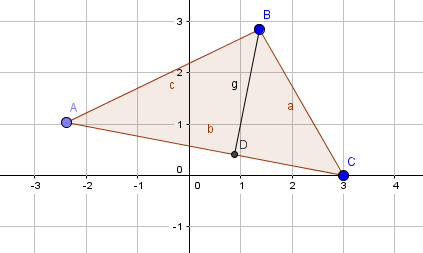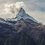# A strange connection in triangles?

Casually I found a relation between the sides of any triangle but I don't know if it is a thing that you can obtain from other theorems or noGiven a triangle $ABC$ I draw the height $BD$ and I call $W$ the value $W=\frac{AB^2+BC^2-AC^2}{2}$ (that is equal to $AB*BC*\cos(\hat{B})$ because of the law of cosines)

Then you can find (I've found this casually, but you can verify easily thanks to GeoGebra or similar programs) $BC^2=DC*AC+W$ and on the other side $AB^2=AD*AC+W$ but I can't explain myself these equalities, could you help me please? Thanks :)Note by Matteo Monzali
5 years, 1 month ago

This discussion board is a place to discuss our Daily Challenges and the math and science related to those challenges. Explanations are more than just a solution — they should explain the steps and thinking strategies that you used to obtain the solution. Comments should further the discussion of math and science.

When posting on Brilliant:

• Use the emojis to react to an explanation, whether you're congratulating a job well done , or just really confused .
• Ask specific questions about the challenge or the steps in somebody's explanation. Well-posed questions can add a lot to the discussion, but posting "I don't understand!" doesn't help anyone.
• Try to contribute something new to the discussion, whether it is an extension, generalization or other idea related to the challenge.

MarkdownAppears as
*italics* or _italics_ italics
**bold** or __bold__ bold
- bulleted- list
• bulleted
• list
1. numbered2. list
1. numbered
2. list
Note: you must add a full line of space before and after lists for them to show up correctly
paragraph 1paragraph 2

paragraph 1

paragraph 2

[example link](https://brilliant.org)example link
> This is a quote
This is a quote
    # I indented these lines
# 4 spaces, and now they show
# up as a code block.

print "hello world"
# I indented these lines
# 4 spaces, and now they show
# up as a code block.

print "hello world"
MathAppears as
Remember to wrap math in $$ ... $$ or $ ... $ to ensure proper formatting.
2 \times 3 $2 \times 3$
2^{34} $2^{34}$
a_{i-1} $a_{i-1}$
\frac{2}{3} $\frac{2}{3}$
\sqrt{2} $\sqrt{2}$
\sum_{i=1}^3 $\sum_{i=1}^3$
\sin \theta $\sin \theta$
\boxed{123} $\boxed{123}$

Sort by:

Check out stewart's theorem.

Staff - 5 years, 1 month ago

Let's put $AB=c \\ BC=a \\ CA=b$

Then the conjectured equations are equivalent to the following equations: $a=b\cos C + c\cos B \\ c=a\cos B + b\cos A$

But these equivalent equations are true for any arbitrary triangles (try proving this).

$\large Q. E. D.$

- 5 years, 1 month ago

Just as a hint, try drawing an altitude from one of the vertices to a side, and then use the two right triangles formed on either side.

- 5 years, 1 month ago

Yes I already know these equations, but I don't understand how they can be equivalent to the ones I wrote (I tried to put them equal but I can't reach a result)

- 5 years, 1 month ago

Notice that $AD=c \cos A$ and $CD=a \cos C$.

- 5 years, 1 month ago